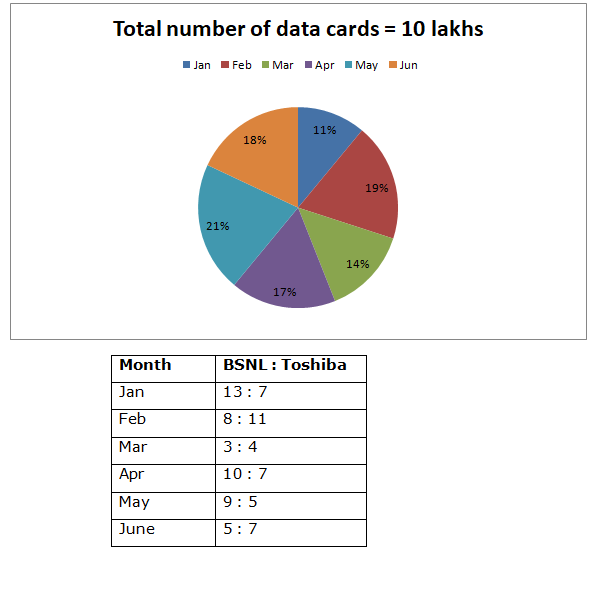# LIC AAO/SBI PO Prelims Quantitative Aptitude Questions 2019 (Day-22)

Dear Aspirants, Our IBPS Guide team is providing new series of Quantitative Aptitude Questions for LIC AAO/SBI PO 2019 so the aspirants can practice it on a daily basis. These questions are framed by our skilled experts after understanding your needs thoroughly. Aspirants can practice these new series questions daily to familiarize with the exact exam pattern and make your preparation effective.

[WpProQuiz 5892]

### Click Here for SBI PO Pre 2019 High-Quality Mocks Exactly on SBI Standard

Directions (Q. 1 – 5) what value should come in place of (?) in the following questions?

1) (1/12) of 3900 + 32 % of 1400 – 122 = ? + 56

a) 573

b) 628

c) 715

d) 477

e) None of these

2) 8 3/5 – 4 ½ + 12 2/5 – 8 ¼ = ? + 3 ½

a) 5 4/5

b) 6 ½

c) 7 ¼

d) 4 ¾

e) None of these

3) 56 of (12/7) of (33/48) of (125/x) = 30

a) 330

b) 275

c) 525

d) 470

e) None of these

4) 272 + 18 % of 1200 – (12/17) of 340 = ?2 + 80

a) 33

b) 52

c) 25

d) 46

e) None of these

5) √[(138 ÷ 6) × (112 ÷ 46) + 122 – 4] = 702 ÷ 9 – ?2

a) 15

b) 22

c) 29

d) 34

e) None of these

Directions (Q. 6 – 10) Study the following information carefully and answer the given questions:

The following pie chart shows the percentage distribution of total number of two company data cards (In lakhs) sold by the shop keeper in six different months and the table shows the ratio of BSNL and Toshiba company data cards sold by the shopkeeper in the given months in a certain state.6) Find the difference between the total number of BSNL data cards sold in the month of Jan and Feb together to that of total number of data cards sold in the month of Apr?

a) 24000

b) 26000

c) 18500

d) 22500

e) None of these

7) Find the ratio between the total number of BSNL data cards sold in the month of Mar to that of total number of Toshiba data cards sold in the month of June?

a) 5 : 9

b) 6 : 11

c) 7 : 12

d) 4 : 7

e) None of these

8) Find the average number of Toshiba data cards sold in the month of Jan, Mar and May together?

a) 64500

b) 72000

c) 68500

d) 74000

e) None of these

9) Total number of Toshiba data cards sold in the month of Feb and Apr together is what percentage more/less than the total number of data cards sold in the month of May?

a) 18.92 % more

b) 14.28 % less

c) 15.36 % more

d) 13.12 % less

e) None of these

10) Find the difference between the average number of data cards sold in the month of Feb and June together to that of the average number of BSNL data cards sold in the month of Apr and May together?

a) 52000

b) 58500

c) 64500

d) 48000

e) None of these

(1/12) of 3900 + 32 % of 1400 – 122 = x + 56

(1/12)*3900 + (32/100)*1400 – 144 – 56 = x

X = 325 + 448 – 144 – 56

X = 573

8 3/5 – 4 ½ + 12 2/5 – 8 ¼ – 3 ½ = x

X = (8 – 4 + 12 – 8 – 3) [(12 – 10 + 8 – 5 – 10)/20]

X = 5 (-5/20) = 5 (-1/4) = 19/4

X = 4 ¾

56*(12/7)*(33/48)*(125/x) = 30

X = 275

272 + 18 % of 1200 – (12/17) of 340 = x2 + 80

729 + (18/100)*1200 – (12/17)*340 – 80 = x2

X2 = 729 + 216 – 240 – 80

X2 = 625

X = 25

√[(138/6) × (112/46) + 122 – 4] = 702 ÷ 9 – x2

√(56 + 144 – 4) = (702/9) – x2

√196 = 78 – x2

X2 = 78 – 14 = 64

X = 8

Directions (6-10):

 Month BSNL : Toshiba BSNL Toshiba Jan 13 : 7 71500 38500 Feb 8 : 11 80000 110000 Mar 3 : 4 60000 80000 Apr 10 : 7 100000 70000 May 9 : 5 13500 75000 June 5 : 7 75000 105000

The total number of BSNL data cards sold in the month of Jan and Feb together

= > 1000000*(11/100)*(13/20) + 1000000*(19/100)*(8/19)

= > 71500 + 80000 = 151500

The total number of data cards sold in the month of Apr

= > 1000000*(17/100) = 170000

Required difference = 170000 – 151500 = 18500

The total number of BSNL data cards sold in the month of Mar

= > 1000000*(14/100)*(3/7)

The total number of Toshiba data cards sold in the month of June

= > 1000000*(18/100)*(7/12)

Required ratio = [1000000*(14/100)*(3/7)] : [1000000*(18/100)*(7/12)]

= > 4 : 7

The total number of Toshiba data cards sold in the month of Jan, Mar and May together

= > 1000000*(11/100)*(7/20) + 1000000*(14/100)*(4/7) + 1000000*(21/100)*(5/14)

= > 38500 + 80000 + 75000 = 193500

Required average = 193500/3 = 64500

Total number of Toshiba data cards sold in the month of Feb and Apr together

= > 1000000*(19/100)*(11/19) + 1000000*(17/100)*(7/17)

= > 110000 + 70000 = 180000

The total number of data cards sold in the month of May

= > 1000000*(21/100) = 210000

Required % = [(210000 – 180000)/210000]*100 = 14.28 % less

The average number of data cards sold in the month of Feb and June together

= > 1000000*(37/100)*(1/2) = 185000

The average number of BSNL data cards sold in the month of Apr and May together

= > [1000000*(17/100)*(10/17) + 1000000*(21/100)*(9/14)]*(1/2)

= > (100000 + 135000)/2 = 117500

Required difference = 185000 – 117500 = 67500